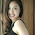## Thursday, June 6, 2013

### Even simpler phylomorphospace

As I mentioned yesterday, I'm working a book chapter on PCM visualization methods. A very small section of that chapter gives an introduction to programming such methods in R. To that end I described a simplified tree plotting function. Here is code for a simplified phylomorphospace plotting function. It needs phytools (and dependencies) and calibrate.

simplePhylomorphospace<-function(tree,x,y){
n<-length(tree\$tip.label)
# get the x & y coordinates of all the tips & nodes
x<-c(x[tree\$tip.label],fastAnc(tree,x))
y<-c(y[tree\$tip.label],fastAnc(tree,y))
# plot tips
plot(x[1:n],y[1:n],cex=1.25,pch=21,bg="black",xlab="x",
ylab="y")
# plot nodes
points(x[1:tree\$Nnode+n],y[1:tree\$Nnode+n],cex=1,pch=21,
bg="black")
# plot lines
apply(tree\$edge,1,function(edge,x,y) lines(x[edge],
y[edge]),x=x,y=y)
# add tip labels (requires 'calibrate')
textxy(x[1:n],y[1:n],tree\$tip.label)
}
(Just seven lines of code, excluding comments.)

Let's see how it works:

> require(phytools)
> require(calibrate)
> source("simplePhylomorphospace.R")
> tree<-pbtree(n=30)
> x<-fastBM(tree)
> y<-fastBM(tree)
> simplePhylomorphospace(tree,x,y)

#### 1 comment:

1.The simple truth is I like this article, the author's writing was very honest, very clever writing skills.

Nersha
www.gofastek.com

Note: due to the very large amount of spam, all comments are now automatically submitted for moderation.